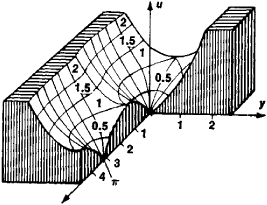# Relief of a Function

The following article is from The Great Soviet Encyclopedia (1979). It might be outdated or ideologically biased.

## Relief of a Function

the surface u = u(x,y) = ǀf(z)ǀ, where f(z) is a complex function of the complex variable z= x + iy. Two systems of curves are usually drawn on this surface: curves of equal modulus, along which ǀf(z)ǀ is constant, and curves of equal argument, along which arg f(z) is constant.Figure 1. Relief of sin z

Some of these curves are provided with numbers giving the values of ǀ f(z)ǀ and arg f(z) on the curves. The modular surface of sin z is shown in Figure 1.

The Great Soviet Encyclopedia, 3rd Edition (1970-1979). © 2010 The Gale Group, Inc. All rights reserved.
Site: Follow: Share:
Open / Close